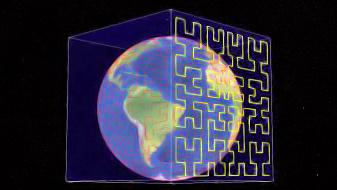Base32对应编码参考图：(上面二进制转换对应的十进制数值)

Geohash其实就是将整个地图或者某个分割所得的区域进行一次划分，由于采用的是base32编码方式，即Geohash中的每一个字母或者数字（如wx4g0e中的w）都是由5bits组成（2^5 = 32，base32），这5bits可以有32中不同的组合（0~31），这样我们可以将整个地图区域分为32个区域，通过00000 ~ 11111来标识这32个区域。第一次对地图划分后的情况如下图所示（每个区域中的编号对应于该区域所对应的编码）

Geohash的0、1串序列是经度0、1序列和纬度0、1序列中的数字交替进行排列的，偶数位对应的序列为经度序列，奇数位对应的序列为纬度序列，在进行第一次划分时，Geohash0、1序列中的前5个bits（11100），那么这5bits中有3bits是表示经度，2bits表示纬度，所以第一次划分时，是将经度划分成8个区段（2^3 = 8），将纬度划分为4个区段（2^2 = 4），这样就形成了32个区域(对应Base32)。如上下图

GeoHash将每一个区域画成一块块矩形块，每个矩形块使用一个字符串表示，当我们需要查询附近的点时，通过自己的坐标计算出一个字符串，根据这个字符串定位到我们所在的矩形块，然后返回这个矩形块中的点。例如 wx4e就包含wx4e0e,也就是说wx4e0ewx4e范围内。

2018年4月21日下午地铁上续更：

11100 11101 00100 01111 10001 10010 00011 00010 01110 10111 11101

28(w)
16+8+4+0+0
1*2^4+1*2^3+1*2^2+0*2^1+0*2^0
11100 --> Base32 -->w

29(x)
16+8+4+0+1
1*2^4+1*2^3+1*2^2+0*2^1+1*2^0
11101 --> Base32 -->x

4(4)
0+0+4+0+0
0*2^4+0*2^3+1*2^2+0*2^1+0^2^0
00100 --> Base32 -->4

15(g)
0+8+4+2+1
0*2^4+1*2^3+1*2^2+1*2^1+1*2^0
01111 --> Base32 -->g

17(j)
16+0+0+0+1
1*2^4+0*2^3+0*2^2+0*2^1+1*2^0
10001 --> Base32 -->j

18(k)
16+0+0+2+0
1*2^4+0*2^3+0*2^2+1*2^1+0*2^0
10010 --> Base32 -->k

3(3)
0+0+0+2+1
0*2^4+0*2^3+0*2^2+1*2^1+1*2^0
00011 --> Base32 -->3

2(2)
0+0+0+2+0
0*2^4+0*2^3+0*2^2+1*2^1+0*2^0
00010 --> Base32 -->2

18(k)
16+0+0+2+0
1*2^4+0*2^3+0*2^2+1*2^1+0*2^0
10010 --> Base32 -->k

14(f)
0+8+4+2+0
0*2^4+1*2^3+1*2^2+1*2^1+0*2^0
01110 --> Base32 -->f

23(r)
16+0+4+2+1
...
10111 --> Base32 -->r

29(x)
16+8+4+0+1
1*2^4+1*2^3+1*2^2+0*2^1+1*2^0
11101 --> Base32 -->x